Courses

# RD Sharma Solutions - Chapter 9 - Linear Equation in One Variable (Part - 2), Class 8, Maths Class 8 Notes | EduRev

## RD Sharma Solutions for Class 8 Mathematics

Created by: Abhishek Kapoor

## Class 8 : RD Sharma Solutions - Chapter 9 - Linear Equation in One Variable (Part - 2), Class 8, Maths Class 8 Notes | EduRev

The document RD Sharma Solutions - Chapter 9 - Linear Equation in One Variable (Part - 2), Class 8, Maths Class 8 Notes | EduRev is a part of the Class 8 Course RD Sharma Solutions for Class 8 Mathematics.
All you need of Class 8 at this link: Class 8

PAGE NO 9.11:

Question 1:

Solve each of the following equation and also check your result in each case: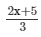= 3x -10= 3x -10

or 2x + 5 = 9 x −30

or 9 x −2x = 5 + 30

or 7x = 35

or  x = 35/7

or  x = 5

Verification:L.H.S. =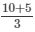= 15/3 = 5

R.H.S. = 15−10 = 5

∴ L.H.S. = R.H.S. for  x = 5.

PAGE NO 9.11:

Question 2:

Solve each of the following equation and also check your result in each case: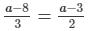or 2a−16 = 3a−9

or 3a−2a = −16 + 9

or a = −7

Verification:

L.H.S. =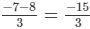= -5

R.H.S. =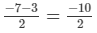= -5
∴ L.H.S. = R.H.S. for a =  −-7

PAGE NO 9.11:

Question 3:

Solve each of the following equation and also check your result in each case: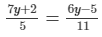or 77y + 22 = 30y−25

or 77y−30y = −25−22

or 47y = −47

or y = −47/47 = −1

Verification:L.H.S. =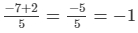R.H.S. =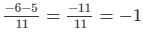∴ L.H.S. = R.H.S. for y =  −-1

PAGE NO 9.11:

Question 4:

Solve each of the following equation and also check your result in each case:

x −2x + 2−16/3x + 5 = 3−7/2x

x −2x + 2−16/3x + 5 = 3−7/2x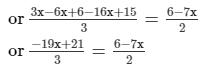or −38 x + 42 = 18−21 x

or −21 x + 38 x = 42−18

or 17x = 24

or  x = 24/17

Check:L.H.S. = 24/17 − 2 × 24/17 + 7 − 16/3 × 24/17 = −33/17

R.H.S. = 3− 7/2 × 24/17 = −33/17

∴ L.H.S. = R.H.S. for  x   =  24/17

PAGE NO 9.11:

Question 5:

Solve each of the following equation and also check your result in each case:

1/2x + 7x −6 = 7x + 1/4

1/2x + 7x −6 = 7x + 1/4

or 1/2x + 7x −7x = 1/4 + 6

or x/2 =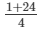or  x/2 = 25/4

or  x = 25/2

Check:L.H.S. = 12×25/2 + 7×25/2-6 = 35/14

R.H.S. = 7×25/2 + 1/4 = 35/14
∴ L.H.S. = R.H.S. for  x   =  25/2

PAGE NO 9.11:

Question 6:

Solve each of the following equation and also check your result in each case:

3/4 x + 4 x = 7/8 + 6 x −6

3/4 x + 4 x = 7/8 + 6 x −6

or 3/4 x −2x = 7/8−6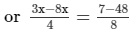or−5 x/ 4 = −41/8

or −40 x = −16/4

or  x = −164/−40 = 41/10

Check:

L.H.S. = 3/4×41/10 + 4×41/10 = 123/40 + 164/10 =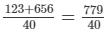R.H.S. = 7/8 + 6×41/10 - 6 = 7/8 + 246/10 - 6 =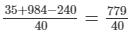∴ L.H.S. = R.H.S. for  x   =  41/10

PAGE NO 9.11:

Question 7:

Solve each of the following equation and also check your result in each case:

7/2 x −5/2 x = 20/3x + 10

7/2 x −5/2 x = 20/3x + 10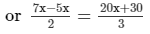or 40 x + 60 = 6 x

or 34 x = −60

or  x = −60/34

= −30/17

Check:

L.H.S. = 7/2×−30/17−5/2× −30/17 = −30/17

R.H.S. = 20/3×−30/17 + 10 = −30/17

∴L.H.S. = R.H.S. for  x = −30/17

PAGE NO 9.11:

Question 8:

Solve each of the following equation and also check your result in each case: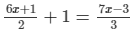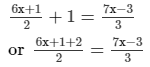or 18 x + 9 = 14 x −6

or 18 x −14 x = −6−9

or 4 x = −15

or  x = −15/4

Check:

L.H.S. =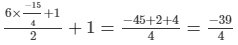R.H.S. =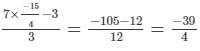∴ L.H.S. = R.H.S. for  x   =  −15/4

PAGE NO 9.11:

Question 9:

Solve each of the following equation and also check your result in each case: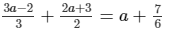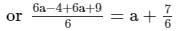or 12a + 5 = 6a + 7

or 6a = 7−5

or a = 2/6 = 1/3

Check:

L.H.S. =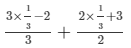= -1/3 + 11/6 = 9/6 = 3/2

R.H.S. = 1/3 + 7/6 = 9/6 = 3/2
∴ L.H.S. = R.H.S. for a  =  1/3

PAGE NO 9.11:

Question 10:

Solve each of the following equation and also check your result in each case: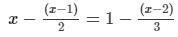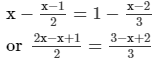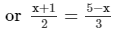or 3x + 3 = 10−2x

or 5 x = 10−3

or  x = 7/5

Check:

L.H.S. =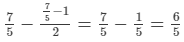R.H.S. =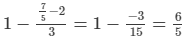∴ L.H.S. = R.H.S. for  x   =  7/5

PAGE NO 9.11:

Question 11:

Solve each of the following equation and also check your result in each case: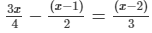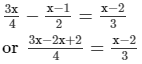or 4 x −8 = 3x + 6

or  x = 14

L.H.S. =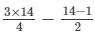= 21/2-13/2 = 8/2 = 4

R.H.S. =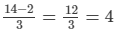∴ L.H.S. = R.H.S. for  x   =  14

PAGE NO 9.11:

Question 12:

Solve each of the following equation and also check your result in each case: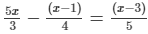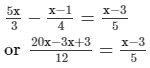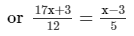or 85 x + 15 = 12x −36

or 73x = −51

or  x = −51/73

Check:L.H.S. =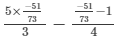= -255/219 - 124292 = -5473

R.H.S. =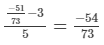∴ L.H.S. = R.H.S. for  x   =  −51/73

PAGE NO 9.11:

Question 13:

Solve each of the following equation and also check your result in each case: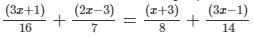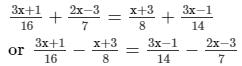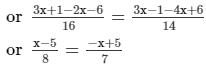or 7x −35 = −8 x + 40

or 15 x = 75

or  x = 75/15 = 5

Check:

L.H.S. =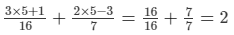R.H.S. =∴ L.H.S. = R.H.S. for  x   = 5

PAGE NO 9.11:

Question 14:

Solve each of the following equation and also check your result in each case: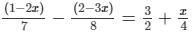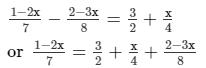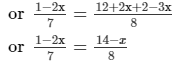or 8−16 x = 98−7x

or −16 x + 7x = 98−8

or  x = −90/9

= −10

Check:L.H.S. =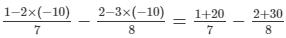= 3-4 = -1

R.H.S. =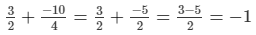∴ L.H.S. = R.H.S. for  x   =  −-10

PAGE NO 9.11:

Question 15:

Solve each of the following equation and also check your result in each case: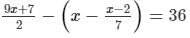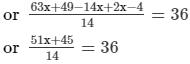or 51 x + 45 = 504

or 51 x = 504−45

or  x = 459/51 = 9

Thus,  x = 9 is the solution of the given equation.

Check:Substituting  x = 9 in the given equation, we get:

L.H.S. =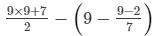= 88/2−9 + 7/7 = 44−9 + 1 = 36

R.H.S. = 36

∴ L.H.S. = R.H.S. for  x = 9.

PAGE NO 9.11:

Question 16:

Solve each of the following equation and also check your result in each case:
0.18(5 x  − 4) = 0.5 x   + 0.8

0.18(5 x −4) = 0.5 x + 0.8

or 0.9 x −0.72 = 0.5 x + 0.8

or 0.9 x −0.5 x = 0.8 + 0.72

or 0.4 x = 1.52

or  x = 1.52/0.4

or  x = 3.8

Thus,  x = 3.8 is the solution of the given equation.

Check:Substituting  x = 3.8 in the given equation, we get:

L.H.S. = 0.18(5×3.8−4) = 0.18×15 = 2.7

R.H.S. = 0.5×3.8 + 0.8 = 2.7

∴ L.H.S. = R.H.S. for  x = 3.8.

PAGE NO 9.11:

Question 17:

Solve each of the following equation and also check your result in each case:

2/3x −3/2x = 1/12

2/3x −3/2x = 1/12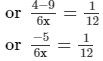or 6 x = −60

or  x = −60/6

or  x = −10

Thus,  x = −10 is the solution of the given equation.

Check:Substituting  x = −10 in the given equation, we get:

L.H.S. =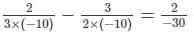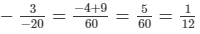R.H.S. = 1/12

∴ L.H.S. = R.H.S. for  x = −10.

PAGE NO 9.11:

Question 18:

Solve each of the following equation and also check your result in each case: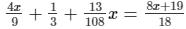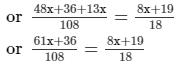or 61 x + 36 = 6(8 x + 19)  [Multiplying both sides by 108]

or 61 x + 36 = 48 x + 114

or 61 x −48 x = 114−36

or 13x = 78

or  x = 78/13

or  x = 6

Thus,  x = 6 is the solution of the given equation.

Check:Substituting  x = 6 in the given equation, we get:

L.H.S. =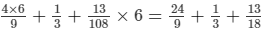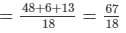R.H.S. =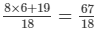∴ L.H.S. = R.H.S. for  x = 6.

PAGE NO 9.12:

Question 19:

Solve each of the following equation and also check your result in each case: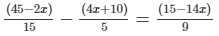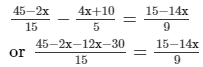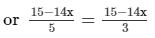[Multiplying both sides by 3]

or 45−42x = 75−70 x   [After cross multiplication]

or 70 x −42x = 75−45

or 28 x = 30

or  x = 30/28

or  x = 15/14

Thus,  x = 15/14 is the solution of the given equation.

Check:Substituting  x = 15/14 in the given equation, we get:

L.H.S. =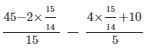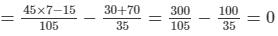R.H.S. =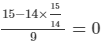∴ L.H.S. = R.H.S. for  x = 15/14

PAGE NO 9.12:

Question 20:

Solve each of the following equation and also check your result in each case: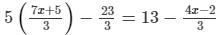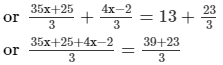or 39 x + 23 = 62 [Multiplying both sides by 3]

or 39 x = 62−23

or  x = 39/39

or  x = 1

Thus,  x = 1 is the solution of the given equation.

Check:Substituting  x = 1 in the given equation, we get:

L.H.S. =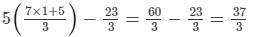R.H.S. =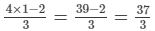∴ L.H.S. = R.H.S. for  x = 1.

PAGE NO 9.12:

Question 21:

Solve each of the following equation and also check your result in each case: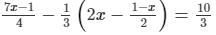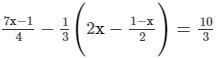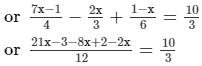or 11 x −1 = 40  [Multiplying both sides by 12]

or 11 x = 40 + 1

or  x = 41/11

Thus,  x = 41/11 is the solution of the given equation.

Check:Substituting  x = 41/11 in the given equation, we get:

L.H.S. =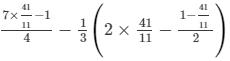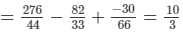R.H.S. = 10/3

∴ L.H.S. = R.H.S. for  x = 41/11

PAGE NO 9.12:

Question 22:

Solve each of the following equation and also check your result in each case: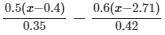= x + 6.1= x + 6.1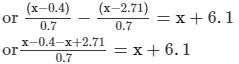or −0.4 + 2.71 = 0.7x + 4.27

or 0.7x = 2.71−0.4−4.27

or  x = −1.96/0.7 = −2.8

Thus,  x = −2.8 is the solution of the given equation.

Check:

Substituting  x = −2.8 in the given equation, we get:

L.H.S. =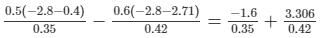= −4.571 + 7.871 = 3.3

R.H.S. = −2.8 + 6.1 = −3.3

∴ L.H.S. = R.H.S. for  x = −2.8

PAGE NO 9.12:

Question 23:

Solve each of the following equation and also check your result in each case:
6.5 x +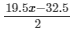= 6.5 x + 13 +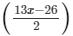6.5 x += 6.5 x + 13 +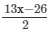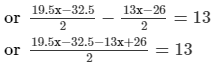or 6.5 x −6.5 = 26 [After cross multiplication]

or 6.5 x = 26 + 6.5

or  x = 32.5/6.5 = 5

Thus,  x = 5 is the solution of the given equation.

Check:

Substituting  x = 5 in the given equation, we get:

L.H.S. = 6.5×5 +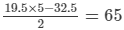R.H.S. = 6.5×5 + 13 +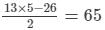∴ L.H.S. = R.H.S. for  x = 5.

PAGE NO 9.12:

Question 24:

Solve each of the following equation and also check your result in each case:
(3x  − 8)(3x   + 2) − (4 x  − 11)(2x   + 1) = ( x  − 3)( x   + 7)

(3x −8)(3x + 2)−(4 x −11)(2x + 1) = ( x −3)( x + 7)

or 9 x² + 6 x −24 x −16−8 x²−4 x + 22x + 11

= x² + 7x −3x −21

or  x²−5 = x² + 4 x −21

or 4 x = −5 + 21

or  x = 16/4 = 4

Thus,  x = 4 is the solution of the given equation.

Check:

Substituting  x = 4 in the given equation, we get:

L.H.S. = (3×4−8)(3×4 + 2)−(4×4−11)(2×4 + 1) = 4×14−5×9 = 11

R.H.S. = (4−3)(4 + 7) = 11

∴ L.H.S. = R.H.S. for  x = 4.

PAGE NO 9.12:

Question 25:

Solve each of the following equation and also check your result in each case:
[(2x   + 3) + ( x   + 5)]2  + [(2x   + 3) − ( x   + 5)]2  = 10 x²  + 92

[(2x + 3) + ( x + 5)]² + [(2x + 3)−( x + 5)]²

= 10 x² + 92

or (3x + 8)² + ( x −2)²

= 10 x² + 92

or 9 x² + 48 x + 64 + x²−4 x + 4

= 10 x² + 92           [ (a + b)² = a² + b² + 2ab  and (a−b)² = a² + b²−2ab ]

or 10 x²−10 x² + 44 x = 92−68

or  x = 24/44

or  x = 6/11

Thus,  x = 6/11 is the solution of the given equation.

Check:

Substituting  x = 6/11 in the given equation, we get:

L.H.S. = [(2 × 6/11 + 3) + (6/11 + 5)]2 + [(2 × 6/11 + 3)−(6/11 + 5)]2

=   [(45/11) + (61/11)]2 + [(45/11)−(61/11)]2

= (106/11)2 + (−16/11)2

= 11492/121

R.H.S. =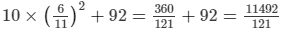∴ L.H.S. = R.H.S. for  x = 6/11

91 docs

,

,

,

,

,

,

,

,

,

,

,

,

,

,

,

,

,

,

,

,

,

,

,

,

,

,

,

;